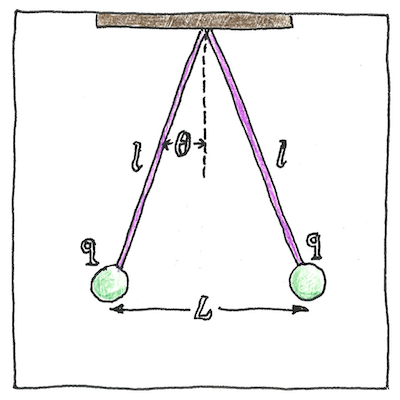# 1785

## The book of science

Tom Sharp

 Charles-Augustin de Coulomb electromagnetism

## Coulomb’s law

• The force between two objects
• that are electrostatically charged
• is directly proportional
• to the magnitude of the charges multiplied together
• and inversely proportional
• to the square of the distance between the objects.
• This law applies
• to any two charged objects with the same polarity,
• to metal spheres
• and to elementary particles.

## History

• Daniel Bernoulli and Alessandro Volta,
• who measured the attractions of capacitor plates,
• and Franz Ulrich Theodor Aepinus
• all supposed the inverse-square law.
• Joseph Priestley,
• who experimented with charged spheres,
• proposed the inverse-square law.
• John Robison
• also measured the force between two charged spheres,
• and announced the inverse-square law.
• Henry Cavendish,
• who later weighed the world with his torsion balance,
• discovered the relationship
• to both distance and amount of charge
• but did not publish.
• Finally, Charles-Augustin de Coulomb,
• after whom the law is named,
• proved the inverse-square law with a torsion balance
• and published the general law.

## Analogies

• Analogous to Isaac Newton’s law
• of universal gravitation
• is Coulomb’s law
• of electrostatic attraction and repulsion,
• which can be used to derive Gauss’s law
• for electric fields,
• which is analogous to Ampère’s circuital law
• for magnetic fields,
• which reminds me of the analogy
• between atoms and solar systems.
• Natural laws are only analogies
• for conditions in the real world
• but we are creatures of analogy;
• all our experiences are analogies.

Coulomb’s law was a significant step in understanding electromagnetic forces. With the work of Volta, Ørsted, Ampère, and Faraday, it led to the invention of electric generators and motors.

See also in The book of science: